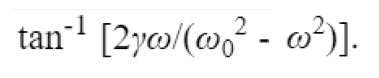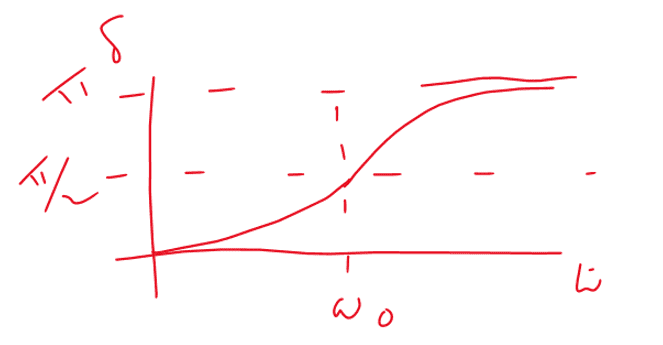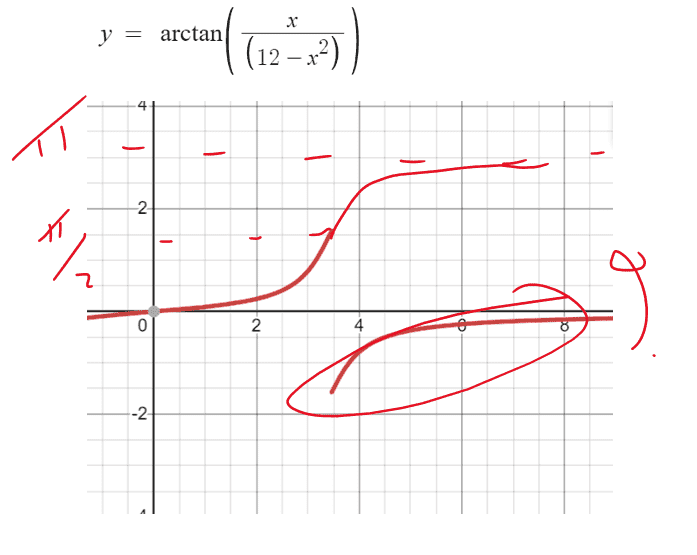# Driven harmonic oscillator

• I
Glenn G
TL;DR Summary
Confused by the Pi rads phase lagThis is an equation I found for the delta phase lag of a driven oscillator. W is the driving angular frequency and Wo is the natural angular frequency of the driven system. Of course this is just a small part of the solution to the differential equation.
Now ... 1) when W is much smaller than Wo the delta phase lag is zero (the driven moves in synch with the driver
2) when W = Wo we have a resonance condition and the phase lag is pi/2
3) when W is much larger than Wo there is a phase lag of pi radians
This can be observed nicely with Barton's pendulae.

The shape of the graph of delta phase lag against w that you find looks like ...But if you plot a graph of a made up function such as ...It seems that if you move the negative portion up you get the correct kind of shape but my issue is that inverse tan only goes from -pi/2 to +pi/2 so the inverse tan of anything, whatever it is never approaches pi.

How can this approach be justified? because it almost seems to me that from the maths that if W is very much bigger than Wo then the arctan is negative so rather that a phase lag, if you are changing sign doesn't this suggest a phase lead? Clearly this is not what happens though when you watch the system oscillating and also it can't be anyway, doesn't make sense.

I would love your input on this ...

Gold Member
2022 Award
This is the usual trouble with this formula to get phase shifts. You shouldn't use the naive arctan formula. It's the same as finding the angle of a point ##(x,y)## (Cartesian coordinates) in polar coordinates. The angle is uniquely defined by the two equations ##\cos \varphi=x/R## and ##\sin \varphi=y/R## with ##R=\sqrt{x^2+y^2}##. Then it's easy to see that the uniquely defined angle is given by
$$\varphi=\begin{cases} \text{sign} y \arccos\left (\frac{x}{R} \right ) & \text{for} \quad y \neq 0, \\ 0 & \text{for} \quad y=0, \quad x>0, \\ \pi &\text{for} \quad y=0, \quad x<0. \end{cases}$$
This angle is in the range ##\varphi \in (-\pi,\pi]##.

•DaveE, topsquark and Glenn G
Glenn G
Thanks Vanhees71 …. So is there a better ‘match’ of formula for the actual shape of the phase characteristics that does go from 0 to pi as W goes from 0 to infinite as in my sketch if the normal arctan isn’t appropriate ?

Gold Member
2022 Award
My convention for the equation of motion is
$$\ddot{x}+2 \gamma \dot{x} + \omega_0^2 x=A \cos(\Omega t)$$
Then amplitude and phase shift for the stationary state are
$$\hat{x}=\frac{A}{\sqrt{(\omega_0^2-\Omega^2)^2+4 \gamma^2 \Omega^2}},$$
$$\varphi=+\arccos \left (\frac{\omega_0^2-\Omega^2}{\sqrt{(\omega_0^2-\Omega^2)^2+4 \gamma^2 \Omega^2}} \right ).$$
This is of course only valid for the case of weak damping, i.e., ##\omega_0>\gamma##.
[EDIT] This is, of course, nonsense. It's valid for any case of damping!

Last edited:
•topsquark and Glenn G
Glenn G
Yay, that looks great for giving the 0, pi/2 and pi relationship for the correct ‘zones’ - was Barton’s pendulae that got me thinking about this. Thanks.

•vanhees71
WernerQH
The relation ## \cot x = 1 / \tan x ## translates to ## \text{arccot}~x = \arctan \frac 1 x ##, and ## \cot x = \tan(\frac \pi 2 - x) ## to ## \text{arccot}~x = \frac \pi 2 - \arctan x ##. So you can use ## \arctan x = \frac \pi 2 - \arctan \frac 1 x ## to rewrite the original puzzling equation as $$\delta = \frac \pi 2 + \arctan {\omega^2 - \omega_0^2 \over 2 \gamma \omega} \ .$$ With multi-valued functions one should be careful not to cross branch cuts inadvertently.

Gold Member
2022 Award
arctan is never a good idea when it comes to find the polar angle in polar coordinates. For that reason most computer languages have a function like atan2 (in Fortran it's ##\phi=\text{atan2}(y,x)## leading to values between ##-\pi## and ##\pi## and with specific rules for ##y=0##).

WernerQH
arctan is never a good idea
Never? The rewritten formula works just fine. :-)

•vanhees71